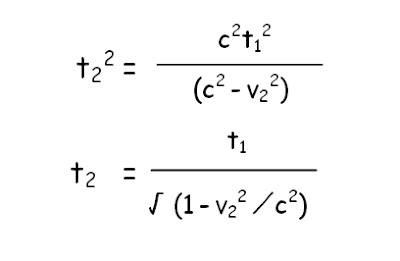## martes, 21 de junio de 2016

### SCIENCE: SPECIAL RELATIVITY

I'm interested in physics, especially in theory of Relativity. As I think is quite difficult to understand it, I will try to explain here why, according to Special Relativity, the constancy of the speed of light leads to a new understanding of time and simultaneity.

Probably the most famous equation of Special Relativity and even of physics

In the following situation there is a train with an uniform movement and velocity v2. There are two observers. One of them is inside the train and the second one is outside the train. Suddenly a bulb is lighted inside the train and its lights go down. The green observer will see that the light arrives to the lower part of the window in the point A in t1, whereas the blue observer will see that the light touches the lower part of the window in the point B in t2. So the light travels a larger distance for the blue observer. The segment AB is the distance travelled by the train between the instants t1 and t2.In the situation shown by the previous figure there are two observers. The blue observer is out of the train. The green observer is inside the train and the relative velocity between this two observers is v2. The first postulate of the special relativity theory states that the reference systems of this two observers are absolutely equivalent. So the phisical laws for each one have to be the same.

Applying the Pitagoras Theorem:According to the second postulate of the special relativity, each observer must measure the same velocity of light, so c=c1=c2The relation between the time measured by the two observers is called gamma factor:

Gamma Factor in Special Relativity

This result is known as Lorentz transformation for time when one observer is at rest (there are another equations for the spatial coordinates).

Lorentz Transformation

For example, if the velocity of the train were half of speed of light, t2 would be 1.15 times t1, so time pass slower for the green observer. In fact, for the green observer the light travels a distance shorter than for the blue observer, so his time will have to pass slower in order to the velocity of light is the same for the two observers. This phenomenon is called time contraction.